# Python Lists Tutorial with Examples

#### View more Tutorials: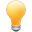Follow us on our fanpages to receive notifications every time there are new articles.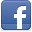Facebook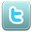Twitter

## 1- Python List

In Python, List is the most flexible data type. It is a sequence of elements, which allows you to remove or add the elements to list and allows you to slice the elements.
In order to write a list, you need to put the elements into a pair of square brackets [] and separate them by the comma. The elements in the list are indexed from the left to right and started from the index 0.
listExample.py
``````

fruitList = ["apple", "apricot", "banana","coconut", "lemen"]

otherList = [100, "one", "two", 3]

print ("Fruit List:")

print (fruitList)

print (" --------------------------- ")

print ("Other List:")

print (otherList)
``````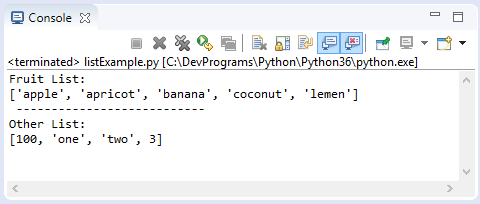## 2- Access the elements of the list

### Access the elements

Use the 'for' loop to access the elements of list:
accessElementExample.py
``````

fruitList = ["apple", "apricot", "banana", "coconut", "lemen", "plum", "pear"]

for fruit in fruitList :

print ("Fruit: ", fruit)
``````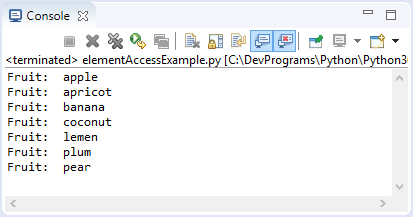### Access via index:

You can also access the elements of list via index. The elements of list are indexed from left to right and started by 0.
indexAccessExample.py
``````
fruitList = ["apple", "apricot", "banana", "coconut", "lemen", "plum", "pear"]

print ( fruitList )

# Element count.
print ("Element count: ", len(fruitList) )

for i in range (0, len(fruitList) ) :

print ("Element at ", i, "= ", fruitList[i] )

# A sub list of elements with index from 1 to 4 (1, 2, 3)
subList = fruitList[1: 4]

# ['apricot', 'banana', 'coconut']
print ("Sub List [1:4] ", subList )
``````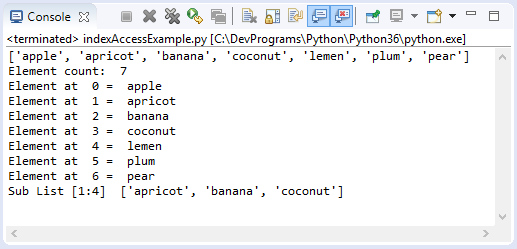You can also access the elements of list by negative index, the elements are indexed from right to left with the value -1, -2,...
indexAccessExample2.py
``````

fruitList = ["apple", "apricot", "banana", "coconut", "lemen", "plum", "pear"]

print ( fruitList )

print ("Element count: ", len(fruitList) )

print ("fruitList[-1]: ", fruitList[-1])
print ("fruitList[-2]: ", fruitList[-2])

subList1 = fruitList[-4: ]

print ("\n")
print ("Sub List fruitList[-4: ] ")
print (subList1)

subList2  = fruitList[-4:-2]

print ("\n")
print ("Sub List fruitList[-4:-2] ")
print (subList2)
``````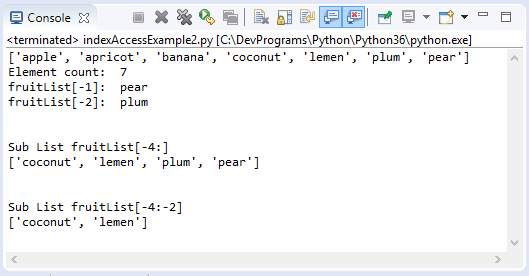## 3- Update List

The example below is a way of List updating by index:
updateListExample.py
``````
years = [1991,1995, 1992]

print ("Years: ", years)

print ("Set years = 2000")

years = 2000

print ("Years: ", years)

print ( years )

print ("Append element 2015, 2016 to list")

# Append an element to the end of the list.
years.append( 2015  )
years.append( 2016  )

print ("Years: ", years)
``````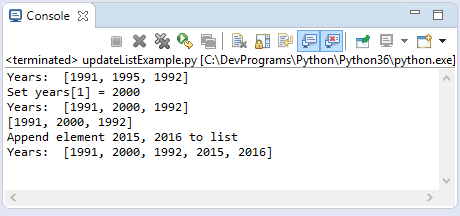You can also update the value to a slice of elements. This is a way helping you to update the multiple elements at a time.
Slice is several consecutive elements in a list.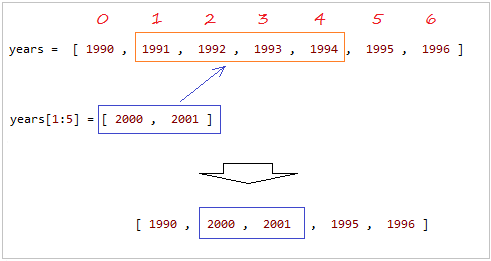sliceUpdateExample.py
``````

years =  [ 1990 ,  1991 ,  1992 ,  1993 ,  1994 ,  1995 ,  1996 ]

print ("Years: ", years)

print ("Update Slice: years[1:5] = [2000, 2001]")

years[1:5] = [ 2000 ,  2001 ]

print ("Years: ", years)
``````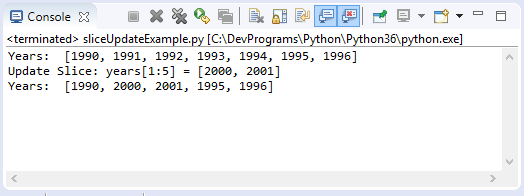## 4- Delete the elements in the list

In order to delete one or more elements in a list, you can use the del statement, or use the remove() method. The example below uses the del statement to delete one or more elements by index.
deleteElementExample.py
``````
years =  [ 1990 ,  1991 ,  1992 ,  1993 ,  1994 ,  1995 ,  1996 ]

print ("Years: ", years)

print ("\n del years")

# Delete element at index = 6.
del years

print ("Years: ", years)

print ("\n del years[1:4]")

# Delete element at index = 1, 2,3
del years[1:4]

print ("Years: ", years)
``````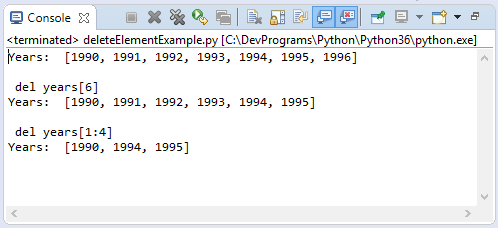The remove(value) method removes the first element in list which has value the same as the value of parameter. The method can throw exception if no element is found to remove.
removeElementExample.py
``````
years =  [ 1990 ,  1991 ,  1992 ,  1993 ,  1994 ,  1993 ,  1993 ]

print ("Years: ", years)

print ("\n years.remove(1993)")

# Remove first occurrence of value.
years.remove(1993)

print ("Years: ", years)
``````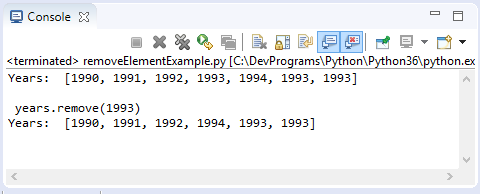## 5- Operators

Like String, List has three operators including +, *, in.
 Operator Description Example + The operator is used to concatenate 2 Lists to create a new List [1, 2, 3[ + ["One","Two"] --> [1, 2, 3, "One", "Two"] * The operator is used to concatenate multiple copies of the same List. And create a new List [1, 2] * 3 --> [1, 2, 1, 2, 1, 2] in Check whether an element is in a List, which returns True or False. "Abc" in ["One", "Abc"] --> True
listOperatorsExample.py
``````
list1 = [1, 2, 3]

list2 = ["One", "Two"]

print ("list1: ", list1)
print ("list2: ", list2)
print ("\n")

list12 = list1 + list2

print ("list1 + list2: ", list12)

list2x3 = list2 * 3

print ("list2 * 3: ", list2x3)

hasThree = "Three" in list2

print ("'Three' in list2? ", hasThree)
``````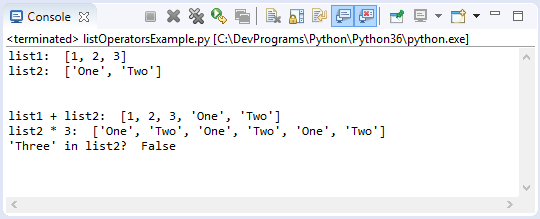## 6- Functions of List

Function Description
cmp(list1, list2) Compares elements of both lists. This function was removed from Python3.
len(list) Gives the total length of the list.
max(list) Returns item from the list with max value.
min(list) Returns item from the list with min value.
list(seq) Converts a tuple into list.
listsFunctionExample.py
``````
list1 = [1991, 1994, 1992]

list2 = [1991, 1994, 2000, 1992]

print ("list1: ", list1)
print ("list2: ", list2)

# Return element count.
print ("len(list1): ", len(list1) )
print ("len(list2): ", len(list2) )

# Maxinum value in the list.
maxValue = max(list1)

print ("Max value of list1: ", maxValue)

# Minimum value in the list
minValue = min(list1)

print ("Min value of list1: ", minValue)

# Tuple
tuple3 = (2001, 2005, 2012)

print ("tuple3: ", tuple3)

# Convert a tuple to a list.
list3 = list (tuple3)

print ("list3: ", list3)
``````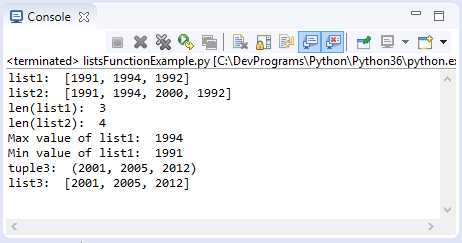See more:

## 7- Methods of List

Method Description
list.append(obj) Appends object obj to list
list.count(obj) Returns count of how many times obj occurs in list
list.extend(seq) Appends the contents of seq to list
list.index(obj) Returns the lowest index in list that obj appears
list.insert(index, obj) Inserts object obj into list at offset index
list.pop([index]) If has index parameter, remove and return the element at index position. Else, remove and return the last element of the list.
list.remove(obj) Removes object obj from list
list.reverse() Reverses objects of list in place
list.sort(key=None, reverse=False) Sorts elements of list by key in parameters
Example:
listMethodsExample.py
``````
years =  [ 1990 ,  1991 ,  1992 ,  1993 ,  1993 ,  1993 ,  1994 ]

print ("Years: ", years)

print ("\n - Reverse the list")

# Reverse the list.
years.reverse()

print ("Years (After reverse): ", years)

aTuple = (2001, 2002, 2003)

print ("\n - Extend: ", aTuple)
years.extend(aTuple)

print ("Years (After extends): ", years)

print ("\n - Append 3000")
years.append(3000)

print ("Years (After appends): ", years)

print ("\n - Remove 1993")
years.remove(1993)

print ("Years (After remove): ", years)

print ("\n - years.pop()")

# Remove last element of list.
lastElement = years.pop()

print ("last element: ", lastElement)

print ("\n")

# Count
print ("years.count(1993): ",years.count(1993) )
``````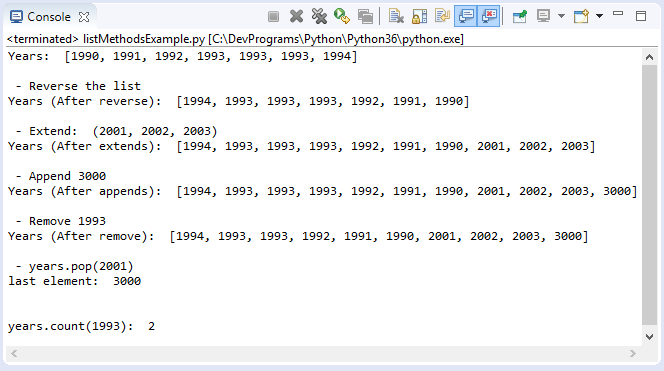See more: